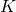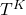# Suppose you are modeling text with a HMM, What is the complexity of finding most the probable sequence of tags or states from a sequence of text using brute force algorithm?

• Assume there are totalstates and letbe the length of the largest sequence.
• Think how we generate text using an hMM. We first have a state sequence and from each state we emit an output. From each state, any word out ofpossible outcomes can be generated. Since there arestates, at each possible state in the sequence, there arechoices of generating a word. Hence, there arepossible sequences
• Therefore, complexity using any brute force algorithm isas we would explore probability of each sequence to find the maximum for most probable one. This is clearly not scalable.